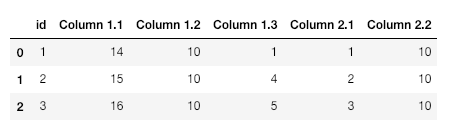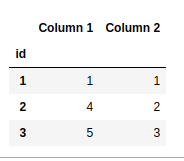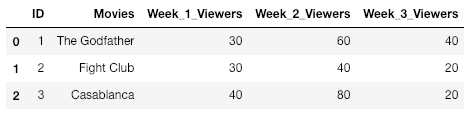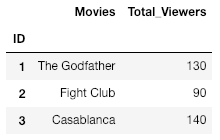GFG App
Open AppBrowser
Continue

# Combining multiple columns in Pandas groupby with dictionary

Let’ see how to combine multiple columns in Pandas using `groupby `with dictionary with the help of different examples.

Example #1:

 `# importing pandas as pd  ` `import` `pandas as pd  ` ` `  `# Creating a dictionary  ` `d ``=` `{``'id'``:[``'1'``, ``'2'``, ``'3'``], ` `     ``'Column 1.1'``:[``14``, ``15``, ``16``], ` `     ``'Column 1.2'``:[``10``, ``10``, ``10``], ` `     ``'Column 1.3'``:[``1``, ``4``, ``5``], ` `     ``'Column 2.1'``:[``1``, ``2``, ``3``], ` `     ``'Column 2.2'``:[``10``, ``10``, ``10``], } ` ` `  `# Converting dictionary into a data-frame  ` `df ``=` `pd.DataFrame(d) ` `print``(df) `

Output:`# Creating the groupby dictionary  ` `groupby_dict ``=` `{``'Column 1.1'``:``'Column 1'``, ` `                ``'Column 1.2'``:``'Column 1'``, ` `                ``'Column 1.3'``:``'Column 1'``, ` `                ``'Column 2.1'``:``'Column 2'``, ` `                ``'Column 2.2'``:``'Column 2'` `} ` ` `  `# Set the index of df as Column 'id' ` `df ``=` `df.set_index(``'id'``) ` ` `  `# Groupby the groupby_dict created above  ` `df ``=` `df.groupby(groupby_dict, axis ``=` `1``).``min``() ` `print``(df) `

Output:Explanation

• Here we have grouped Column 1.1, Column 1.2 and Column 1.3 into Column 1 and Column 2.1, Column 2.2 into Column 2.
• Notice that the output in each column is the min value of each row of the columns grouped together. i.e in Column 1, value of first row is the minimum value of Column 1.1 Row 1, Column 1.2 Row 1 and Column 1.3 Row 1.

Example #2:

 `# importing pandas as pd  ` `import` `pandas as pd  ` ` `  `# Create dictionary with data  ` `dict` `=` `{ ` `    ``"ID"``:[``1``, ``2``, ``3``], ` `    ``"Movies"``:[``"The Godfather"``, ``"Fight Club"``, ``"Casablanca"``], ` `    ``"Week_1_Viewers"``:[``30``, ``30``, ``40``], ` `    ``"Week_2_Viewers"``:[``60``, ``40``, ``80``], ` `    ``"Week_3_Viewers"``:[``40``, ``20``, ``20``] }; ` ` `  `# Convert dictionary to dataframe ` `df ``=` `pd.DataFrame(``dict``); ` `print``(df) `

Output:`# Create the groupby_dict  ` `groupby_dict ``=` `{``"Week_1_Viewers"``:``"Total_Viewers"``, ` `           ``"Week_2_Viewers"``:``"Total_Viewers"``, ` `           ``"Week_3_Viewers"``:``"Total_Viewers"``, ` `           ``"Movies"``:``"Movies"` `} ` ` `  `df ``=` `df.set_index(``'ID'``) ` `df ``=` `df.groupby(groupby_dict, axis ``=` `1``).``sum``() ` `print``(df) `

Output:Explanation:

• Here, notice that even though ‘Movies’ isn’t being merged into another column it still has to be present in the groupby_dict, else it won’t be in the final dataframe.
• To calculate the Total_Viewers we have used the .sum() function which sums up all the values of the respective rows.

My Personal Notes arrow_drop_up